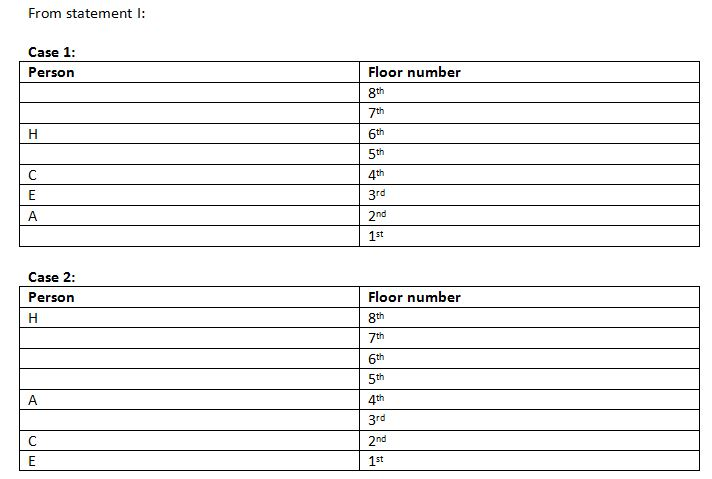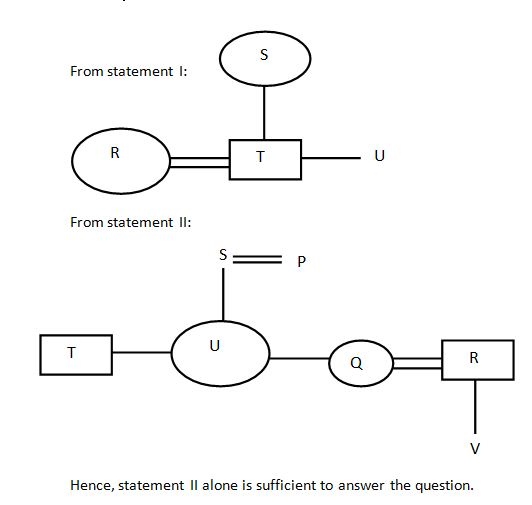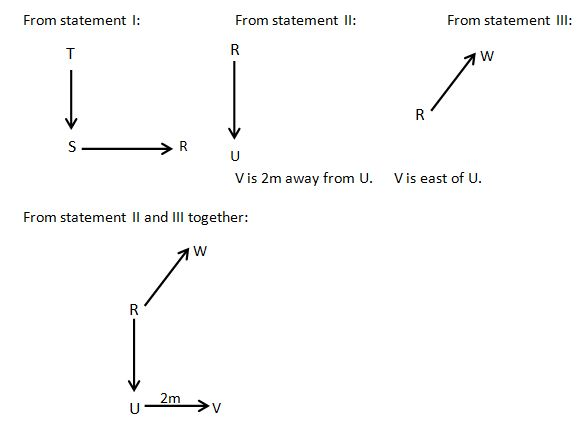# Reasoning Questions: Data Sufficiency Set 18

Welcome to Online Quantitative Aptitude section in AffairsCloud.com. Here we are creating question sample From DATA SUFFICIENCY  which is BASED ON Bank EXAMS 2019 !!!

(Directions 1 – 10): Study the following information and answer the questions given below:

1. Among persons A, B, C, D, I and J which is the eldest?
I. C is elder than only two persons. B is elder than A but not the eldest. I is elder than only J.
II. A is younger than only two persons. I is elder than J but younger than C. C is younger than A. B is younger than D.
1) The data in statement II alone are sufficient to answer the question, while the data in statement I alone are not sufficient to answer the question
2) The data even in both statements I and II together are not sufficient to answer the question
3) The data either in statement I alone or in statement II alone are sufficient to answer the question
4) The data in statement I alone are sufficient to answer the question, while the data in statement II alone are not sufficient to answer the question
5) The data in both statements I and II together are necessary to answer the question
Answer & Explanation
Answer – 3) The data either in statement I alone or in statement II alone are sufficient to answer the question
Explanation :
From statement I:
C is elder than only two persons. B > A but B is not the eldest. I is elder than J only.
D > B > A > C > I > J
From statement II:
A is younger than only two persons. I > J, C > I. A > C. D > B
D > B > A > C > I > J
Hence, the data either in statement I alone or in statement II alone are sufficient to answer the question.
2. Eight persons A, B, C, D, E, F, G and H are staying on an eight storey building. Bottom floor is numbered one and top floor is numbered eight. Who among the following lives on top floor?
I. A lives on an even numbered floor but not on top floor. Three floors are between A and H. The number of person above H is same as below E. C lives just above E but not on top floor.
II. Two floors are between A and D. One floor is between D and F. G lives two floors above E. A live just above G.
1) The data in statement II alone are sufficient to answer the question, while the data in statement I alone are not sufficient to answer the question
2) The data even in both statements I and II together are not sufficient to answer the question
3) The data either in statement I alone or in statement II alone are sufficient to answer the question
4) The data in statement I alone are sufficient to answer the question, while the data in statement II alone are not sufficient to answer the question
5) The data in both statements I and II together are necessary to answer the question
Answer & Explanation
Answer – 5) The data in both statements I and II together are necessary to answer the question
Explanation :3. How many people are standing there in a straight line, all of them facing the north?
I) Only one person stands to the left of S. Only two person stand between S and D. D stand to immediate right to of M. M stand exactly in the middle of the line.
II) Only three people stand to the left of M. Only two person stand between M and P. P stand at the extreme right end of the line.
1) The data in statement II alone are sufficient to answer the question, while the data in statement I alone are not sufficient to answer the question
2) The data even in both statements I and II together are not sufficient to answer the question
3) The data either in statement I alone or in statement II alone are sufficient to answer the question
4) The data in statement I alone are sufficient to answer the question, while the data in statement II alone are not sufficient to answer the question
5) The data in both statements I and II together are necessary to answer the question
Answer & Explanation
Answer – 3) The data either in statement I alone or in statement II alone are sufficient to answer the question
Explanation :

4. Seven persons P, Q, R, S, T, U and V are belonging to the same family. It is a three-generation family and two married couples are there in the family. How is U related to V?
I. T is brother of U and son of S. S is mother-in-law of R and grandmother of V. R is not married to U.
II. U is daughter of S and sister of Q. T is son of P and brother-in-law of R. R is father of V. R is not married to U.
1) The data in statement II alone are sufficient to answer the question, while the data in statement I alone are not sufficient to answer the question
2) The data even in both statements I and II together are not sufficient to answer the question
3) The data either in statement I alone or in statement II alone are sufficient to answer the question
4) The data in statement I alone are sufficient to answer the question, while the data in statement II alone are not sufficient to answer the question
5) The data in both statements I and II together are necessary to answer the question
Answer & Explanation
Answer – 1) The data in statement II alone are sufficient to answer the question, while the data in statement I alone are not sufficient to answer the question
Explanation :5. Among six people – A, B, C, D, E and F each having a different weight who is the second heaviest?
I. Only two people are lighter than D. Only two people are heavier than A. E is lighter than C. B is heavier than only F.
II. Only three people are lighter than A. B is lighter than D, but not the lightest. C is the heaviest amongst them.
1) The data in statement II alone are sufficient to answer the question, while the data in statement I alone are not sufficient to answer the question
2) The data even in both statements I and II together are not sufficient to answer the question
3) The data either in statement I alone or in statement II alone are sufficient to answer the question
4) The data in statement I alone are sufficient to answer the question, while the data in statement II alone are not sufficient to answer the question
5) The data in both statements I and II together are necessary to answer the question
Answer & Explanation
Answer – 1) The data in statement II alone are sufficient to answer the question, while the data in statement I alone are not sufficient to answer the question
Explanation :
From statement I:
Only two people are lighter than D. Only two people are heavier than A. C > E. B > F.
C > E > A > D > B > F

From statement II:
Only three people are lighter than A. D > B and B is not the lightest. C is not the heaviest.

Hence, statement I alone is sufficient to answer the question.

6. Eight persons M, N, O, P, Q, R, S and T are sitting in a straight line and the persons are sitting at the both extreme ends are facing south and rest are facing north direction. Who among the following sits at the left end?
I. M is 3rd from the right end. Two persons are sitting between M and S. N is 3rd to the left of T. T is not neighbour of M or S.
II. Two persons are sitting between P and Q. O is 3rd to the right of Q. M is 3rd from the right end.
1) The data in statement II alone are sufficient to answer the question, while the data in statement I alone are not sufficient to answer the question
2) The data even in both statements I and II together are not sufficient to answer the question
3) The data either in statement I alone or in statement II alone are sufficient to answer the question
4) The data in statement I alone are sufficient to answer the question, while the data in statement II alone are not sufficient to answer the question
5) The data in both statements I and II together are necessary to answer the question
Answer & Explanation
Answer – 4) The data in statement I alone are sufficient to answer the question, while the data in statement II alone are not sufficient to answer the question
Explanation :

7. In which month (of the same year) did Ram visit Goa?
I. Ram’s mother correctly remembers that Ram visit Goa after June, but before October and that month had less than 31 days.
II. Ram’s father correctly remembers that Ram visit Goa after August, but before December and the month had only 30 days.
1) The data in statement II alone are sufficient to answer the question, while the data in statement I alone are not sufficient to answer the question
2) The data even in both statements I and II together are not sufficient to answer the question
3) The data either in statement I alone or in statement II alone are sufficient to answer the question
4) The data in statement I alone are sufficient to answer the question, while the data in statement II alone are not sufficient to answer the question
5) The data in both statements I and II together are necessary to answer the question
Answer & Explanation
Answer – 4) The data in statement I alone are sufficient to answer the question, while the data in statement II alone are not sufficient to answer the question
Explanation :
From statement I:
Ram visit Goa in the month of September.

From statement II:
Ram visit Goa in the month of September or November.

Hence, statement I alone is sufficient to answer the question.

8. Which among the five employees A, B, C, D and E is the best?
I. D is better than E, A and C but not as good as B.
II. D is better than C but not as good as B which is better than E.
1) The data in statement II alone are sufficient to answer the question, while the data in statement I alone are not sufficient to answer the question
2) The data even in both statements I and II together are not sufficient to answer the question
3) The data either in statement I alone or in statement II alone are sufficient to answer the question
4) The data in statement I alone are sufficient to answer the question, while the data in statement II alone are not sufficient to answer the question
5) The data in both statements I and II together are necessary to answer the question
Answer & Explanation
Answer – 4) The data in statement I alone are sufficient to answer the question, while the data in statement II alone are not sufficient to answer the question
Explanation :
From statement I:
D > E, A and C, B > D
B > D > E, A and C

From statement II:
D > C, B > D, B > E

Hence, statement I alone is sufficient to answer the question.

9. What is the direction of car R with respect to car V?
I. R is to the east of S and to the south of T.
II. U is to the south of R and V is 2m away from U.
III. W is to the north east of R and V is to the east of U.
1) Only III
2) Only I and II
3) Only II and III
4) Question cannot be answered even with all I, II & III
5) None of these
Answer & Explanation
Answer – 3) Only II and III
Explanation :10. What was the grand total of Team India in today’s match?
I. Madhav correctly remembers that team India scored a grand total of above 82 but below 91.
II. Akash correctly remembers that team India scored above 77 and below 84.
1) The data in statement II alone are sufficient to answer the question, while the data in statement I alone are not sufficient to answer the question
2) The data even in both statements I and II together are not sufficient to answer the question
3) The data either in statement I alone or in statement II alone are sufficient to answer the question
4) The data in statement I alone are sufficient to answer the question, while the data in statement II alone are not sufficient to answer the question
5) The data in both statements I and II together are necessary to answer the question
Answer & Explanation
Answer – 5) The data in both statements I and II together are necessary to answer the question
Explanation :
From statement I:
Team India scores either 83, 84, 85, 86, 87, 88, 89 or 90.

From statement II:
Team India either scores 78, 79, 80, 81, 82 or 83.

From statement I and II together:
Team India scores 83 runs.

AffairsCloud Recommends IBPSGuide Mock Test